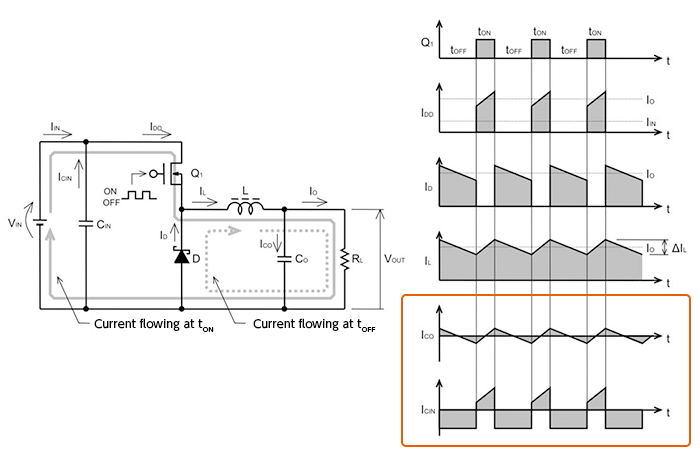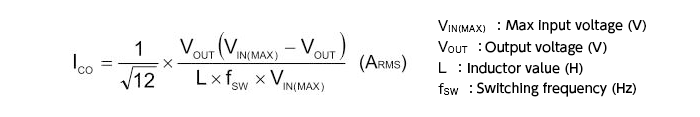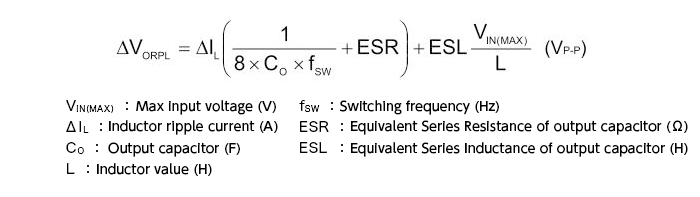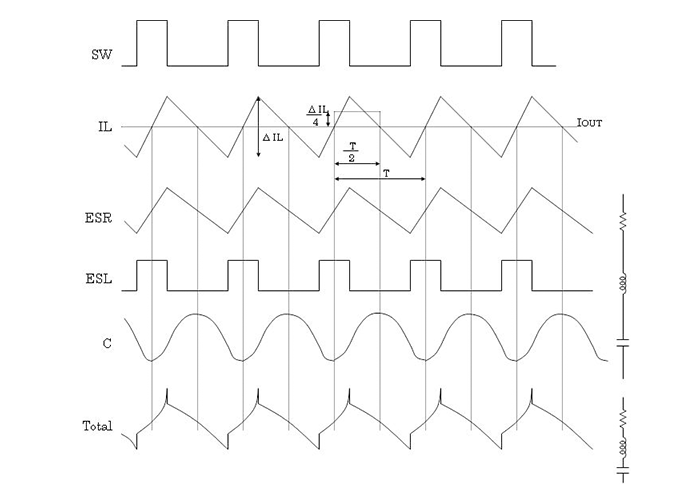Technical Information Site of Power Supply Design

2017.04.27 DC/DC

# Selection of Output Capacitors

Selection of Inductors and Capacitors for DC/DC Converters

In succession to selection of inductors, we turn to a discussion of capacitor selection. Capacitors that are essential for a step-down DC/DC converter include output capacitors and input capacitors. We begin by explaining output capacitors. Similarly to inductor selection, the choice of capacitor is also very important. Selection methods, recommended types and the like are essentially described in data sheets and related supporting materials, and these should be consulted.

Roles of input and output capacitors

First, in order to understand the roles of input capacitors and output capacitors, we review the current flows in a step-down DC/DC converter. By understanding the differences in the currents flowing in each capacitor, we will see what kinds of capacitors should be selected in each case.The same diagrams were used in “Basic Operation of Step-down Converters” in the waveforms enclosed in the rectangle, the upper waveform ICO and lower waveform ICIN are the current waveforms of the output and input capacitors respectively. The input capacitor is charged by VIN, and when the transistor Q1 turns on, discharges a current that becomes the switch current IDD. Comparatively high currents flow suddenly and repeatedly. The output capacitor is repeatedly charged and discharged according to the output ripple voltage, which is centered on the output voltage.

Selection of the output capacitor

From here, we discuss output capacitors. The following three factors are important when selecting the output capacitor.

1）Rated voltage

2）Rated ripple current

3）ESR (equivalent series resistance)

Of course the voltage and ripple current applied to a capacitor must be below the maximum ratings for the capacitor. The ESR is an important parameter that determines the output ripple voltage associated with the inductor current, and must be studied carefully.

The ripple current of an output capacitor is a sawtooth wave, as indicated by ICO in the waveform diagram above; its effective value can be represented by the following equation.The output ripple voltage is represented by the following equation as the composite waveform resulting from the ripple component ΔIL of the inductor current IL in the above diagram, and the voltage occurring due to the capacitance, ESR, and ESL of the output capacitor.When illustrated as waveforms, we obtain the following.The ripple component ΔIL of the inductor current generated by switching causes a ripple voltage that is simply proportional to the ESR, and the ESL generates a square-wave voltage. The electrostatic capacitance component is combined with these to result in the final output ripple voltage waveform, shown at the bottom.

When representing the output ripple voltage, the following equation is used. Because the phases of the ripple due to the capacitor and the ripple due to the ESR are shifted, the two cannot simply be added together, but the following equation is often used to estimate the worst case value of the ripple voltage.From this equation we see that in order to reduce the output ripple voltage, we should lower the ESR, increase the capacitance of the output capacitor, raise the switching frequency and reduce IL to the minimum necessary level.

In recent years, there appears to be a trend toward increased use of multilayer ceramic capacitors as output capacitors. Ceramic capacitors have extremely low ESR and ESL values, and so observed ripples originate almost entirely in the capacitance of the capacitor.

#### Key Points:

・When selecting an output capacitor, the rated voltage, rated ripple current, and ESR are important parameters.

・In addition to smoothing and regulation, output capacitors are also closely related to the output ripple voltage.